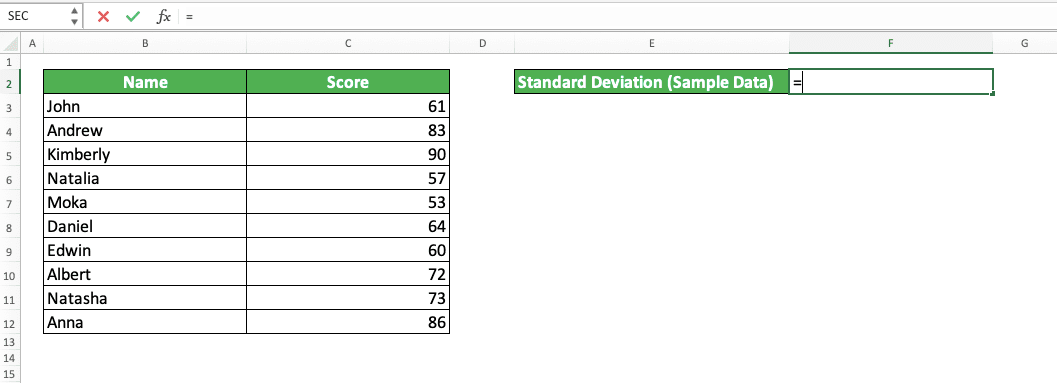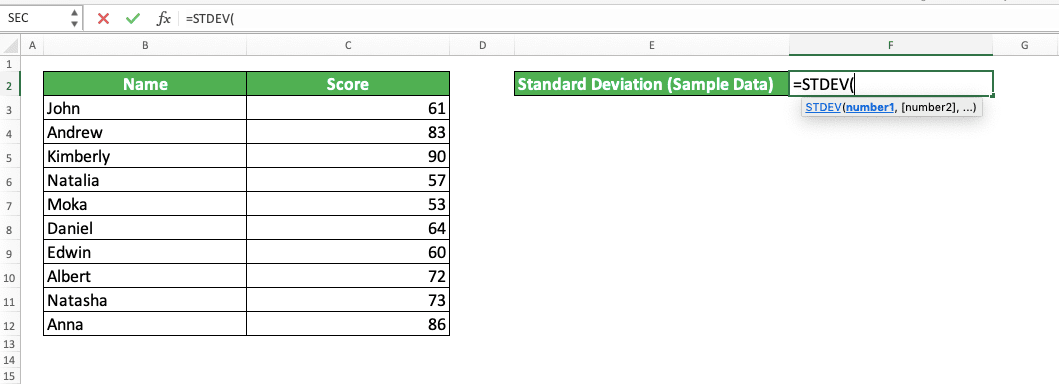How to Use the STDEV Formula in Excel: Functions, Examples and Writing Steps - Compute Expert

# How to Use the STDEV Formula in Excel: Functions, Examples and Writing Steps

Home >> Excel Tutorials from Compute Expert >> Excel Formulas List >> How to Use the STDEV Formula in Excel: Functions, Examples and Writing Steps

In this tutorial, you will learn how to use the STDEV formula in excel completely.

When working with numbers in excel, we may sometimes need to calculate the standard deviation of our sample data numbers. If we know how to use STDEV, then we can calculate that standard deviation easily.

Want to know more about STDEV and master the way to use this function in excel? Read this tutorial until its last part!

Disclaimer: This post may contain affiliate links from which we earn commission from qualifying purchases/actions at no additional cost for you. Learn more

## What is the STDEV Formula in Excel?

STDEV is an excel function that helps you to calculate the standard deviation of your sample data numbers.

## STDEV Function in Excel

You can use STDEV to get the standard deviation of your sample data numbers in excel.

## STDEV Result

The result from STDEV is a number that represents the standard deviation of the sample data numbers you input into it.

## Excel Version from Which We Can Start Using STDEV

You can start using STDEV since excel 2003.

## The Way to Write It and Its Inputs

Here is the general writing form of STDEV in excel.

= STDEV ( number1 , [ number2 ] , … )

You only need to input your sample data numbers to STDEV to get your standard deviation. You can input them by typing them directly in STDEV or by using cell or cell range coordinates. However, we usually only input one cell range to STDEV that contains all of our sample data numbers.

If you give more than one inputs to STDEV, you must separate them by using comma signs ( , ).

## Example of Its Usage and Result

Here is the implementation example of STDEV in excel.As you can see, we just need to input the numbers we want to calculate the standard deviation to STDEV. However, we need to make sure that the numbers we input are samples so we don’t get the wrong result.

## Writing Steps

After we discussed the STDEV writing form, inputs, and implementation example, now let’s discuss its writing steps. As you only need to input your sample data numbers to STDEV, these writing steps are simple to understand.

1. Type an equal sign ( = ) in the cell where you want to put the standard deviation of your sample data numbers2. Type STDEV (can be with large and small letters) and an open bracket sign after =3. Input the sample data numbers you want to get your standard deviation from. You can input them by typing them directly or by using cell or cell range coordinates.

If you give more than one inputs, separate them with comma signs4. Type a close bracket sign5. Press Enter
6. Done!## Other Standard Deviation Functions in Excel

Besides STDEV, there are other functions in excel you can use to calculate standard deviation from your numbers. Here is a table that lists them and their differences.

Excel FunctionCalculates Standard Deviation from…Treatment on Logic Values and Text (If They Are in the Cell/Cell Range We Input)
STDEV.Ssample dataignore
STDEVPpopulation dataignore
STDEV.Ppopulation dataignore
STDEVAsample dataassume FALSE and text as 0 and TRUE as 1
STDEVPApopulation dataassume FALSE and text as 0 and TRUE as 1

As with STDEV.S, STDEVP, and STDEV.P, STDEV ignores logic values and text inputs (if they are in a cell/a cell range).

You can use STDEV.S and STDEV.P since excel 2010 while you can use the other functions since excel 2003.

You might notice that STDEV-STDEV.S and STDEVP-STDEV.P pairs function the same way. Officially, Microsoft recommends that you use STDEV.S and STDEV.P since they are the updated versions of STDEV and STDEV.P (Microsoft retains STDEV and STDEV.P for backward compatibility). However, those functions don’t seem to have much difference between them.

## Exercise

After you learned how to use the STDEV formula in excel, now let’s sharpen your understanding by doing the following exercise.

### Questions

Give your answer by using STDEV in the appropriate gray-colored cell according to the question number.
1. What is the standard deviation of these sample answer choice numbers?
2. What is the standard deviation if we add 3 and 2 to our sample?
3. What is the standard deviation if we add 5, 4,1, 3, and 2 to our sample?

• You can give up to 255 different inputs to STDEV
• STDEV will consider logic values and text if you type them directly into it. It will treat FALSE as 1, TRUE as 0, and text as something that will make it produces a #VALUE error

Related tutorials you should learn too:

Get updated excel info from Compute Expert by registering your email. It's free!